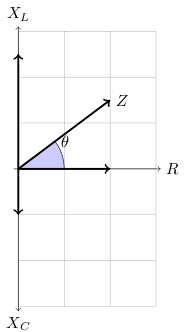# Impedance

Impedance is the opposition to an ac current.

## Series circuit

In a series circuit, impedance is the vector sum of resistance and reactance (capacitive reactance, inductive reactance). Let `Z` be the impedance, `V` the peak or root mean square (RMS) voltage as a vector, and `I` be the peak or RMS current as a vector. Then

```	```
Z = V/I
```
```

As in the notes for LCR series circuit, let the peak or RMS voltage through a resistor be `VR`, the peak or RMS voltage across an inductor be `VL`, and the peak or RMS voltage across the capacitor be `VC`. The total peak or RMS voltage, `VT`, can calculated as shown below

```	```
|VT| = √(|VR|2+|VL-VC|2)
|VT|/|I| = √(|VR|2/|I|2+|VL/I-VC/I|2)
```
```

From the notes on capacitive reactance, `XC`, inductive reactance, `XL`, and Ohm's law, we have

```	```
XC = VC/I
XL = VL/I
R = VR/I
```
```

So

```	```
|VT|/|I| = √(|VR|2/|I|2+|VL/I-VC/I|2)
= √(R2+|XL-XC|2)
|Z|      = √(R2+|XL-XC|2)
```
```

Note that using complex numbers, we can write:

```	```
V = VR+j(VL-VC)
Z = V/I
= VR/I+j(VL-VC)/I
= R+j(XL-XC)
= R +j(ωL-1/(ωC))
```
```

For the phase, i.e. difference between the maximum current and voltage, we have:```	```
tan θ = (XL-XC)/R
```
```

In a RC series circuit, `XL=0`, gives

```	```
tan θ = -XC/R
= -1/(RωC)
```
```

In a RL series circuit, `XC=0`, gives

```	```
tan θ = XL/R
= ωL/R
```
```

A LCR circuit is

• capacitively reactive if `XC>XL`
• inductively reactive if `XC<XL`
• resonant if `XC=XL`

## References

Fischer-Cripps. A.C., The Electronics Companion. Institute of Physics, 2005.